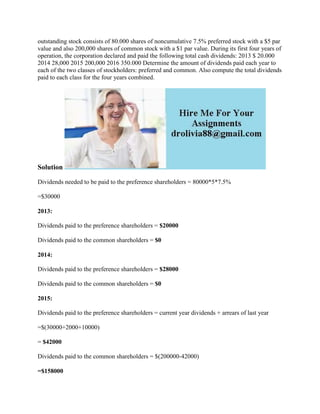Diese Präsentation wurde erfolgreich gemeldet.

# outstanding stock consists of 80-000 shares of noncumulative 7-5- pref.docx

Anzeige
Anzeige
Anzeige
Anzeige
Anzeige
Anzeige
Anzeige
Anzeige
Anzeige
Anzeige
Anzeige×

1 von 2 Anzeige

# outstanding stock consists of 80-000 shares of noncumulative 7-5- pref.docx

outstanding stock consists of 80.000 shares of noncumulative 7.5% preferred stock with a \$5 par value and also 200,000 shares of common stock with a \$1 par value. During its first four years of operation, the corporation declared and paid the following total cash dividends: 2013 \$ 20.000 2014 28,000 2015 200,000 2016 350.000 Determine the amount of dividends paid each year to each of the two classes of stockholders: preferred and common. Also compute the total dividends paid to each class for the four years combined.
Solution
Dividends needed to be paid to the preference shareholders = 80000*5*7.5%
=\$30000
2013:
Dividends paid to the preference shareholders = \$20000
Dividends paid to the common shareholders = \$0
2014:
Dividends paid to the preference shareholders = \$28000
Dividends paid to the common shareholders = \$0
2015:
Dividends paid to the preference shareholders = current year dividends + arrears of last year
=\$(30000+2000+10000)
= \$42000
Dividends paid to the common shareholders = \$(200000-42000)
=\$158000
2016:
Dividends paid to the preference shareholders = \$30000
Dividends paid to the common shareholders = \$(350000-30000)
=\$320000
.

outstanding stock consists of 80.000 shares of noncumulative 7.5% preferred stock with a \$5 par value and also 200,000 shares of common stock with a \$1 par value. During its first four years of operation, the corporation declared and paid the following total cash dividends: 2013 \$ 20.000 2014 28,000 2015 200,000 2016 350.000 Determine the amount of dividends paid each year to each of the two classes of stockholders: preferred and common. Also compute the total dividends paid to each class for the four years combined.
Solution
Dividends needed to be paid to the preference shareholders = 80000*5*7.5%
=\$30000
2013:
Dividends paid to the preference shareholders = \$20000
Dividends paid to the common shareholders = \$0
2014:
Dividends paid to the preference shareholders = \$28000
Dividends paid to the common shareholders = \$0
2015:
Dividends paid to the preference shareholders = current year dividends + arrears of last year
=\$(30000+2000+10000)
= \$42000
Dividends paid to the common shareholders = \$(200000-42000)
=\$158000
2016:
Dividends paid to the preference shareholders = \$30000
Dividends paid to the common shareholders = \$(350000-30000)
=\$320000
.

Anzeige
Anzeige

### outstanding stock consists of 80-000 shares of noncumulative 7-5- pref.docx

1. 1. outstanding stock consists of 80.000 shares of noncumulative 7.5% preferred stock with a \$5 par value and also 200,000 shares of common stock with a \$1 par value. During its first four years of operation, the corporation declared and paid the following total cash dividends: 2013 \$ 20.000 2014 28,000 2015 200,000 2016 350.000 Determine the amount of dividends paid each year to each of the two classes of stockholders: preferred and common. Also compute the total dividends paid to each class for the four years combined. Solution Dividends needed to be paid to the preference shareholders = 80000*5*7.5% =\$30000 2013: Dividends paid to the preference shareholders = \$20000 Dividends paid to the common shareholders = \$0 2014: Dividends paid to the preference shareholders = \$28000 Dividends paid to the common shareholders = \$0 2015: Dividends paid to the preference shareholders = current year dividends + arrears of last year =\$(30000+2000+10000) = \$42000 Dividends paid to the common shareholders = \$(200000-42000) =\$158000
2. 2. 2016: Dividends paid to the preference shareholders = \$30000 Dividends paid to the common shareholders = \$(350000-30000) =\$320000